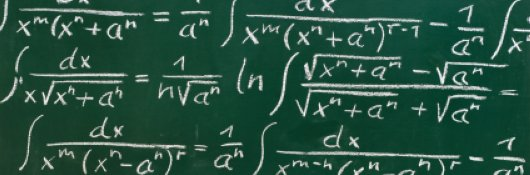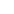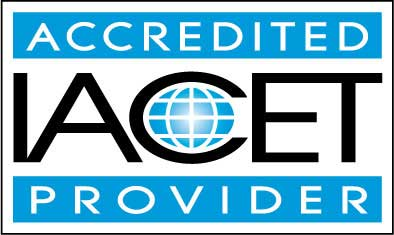# Course Syllabus## Course Description

Large sets of numbers can be daunting, and characterizing them in a few words or numbers can be even more daunting. This course considers how to take data sets--whether large or small--and describe them using a few numbers (descriptive statistics). This, however, is only a small portion of the course.

The majority of the course is dedicated to reaching statistically justified conclusions on the basis of these descriptive statistics. For instance, does the average value of one data set deviate from another in what might be called a "statistically significant" manner? To this end, the course covers cross tabulation of data (including the chi-square test), correlation, linear regression, Student's t-tests, analysis of variance (ANOVA), repeated measures analysis, and factor analysis.

Although the course does not always provide rigorous mathematical justifications for every aspect of the statistical tools and theory discussed therein, it does provide common-sense explanations of many of these aspects, and it includes numerous real-world examples to illustrate the use of these tools.

Thus, this course teaches students to take sets of data, describe them using a few numbers (including the mean, variance, and skewness), and then reach statistically justifiable conclusions about those data sets. Students should come away from the course with confidence in their ability to tackle basic applied statistics problems and with the fundamental knowledge needed to learn more in-depth statistical theory.

• Completely Online
• Self-Paced
• Printable Lessons
• Full HD Video• 6 Months to Complete
• Start Anytime
• PC & Mac Compatible
• Android & iOS Friendly
• Accredited CEUs## Learning Outcomes

By successfully completing this course, students will be able to:
• Define descriptive statistics.
• Demonstrate statistics and the use frequencies and how to solve these problems.
• Identify multivariate data.
• Demonstration Cross Tabulation problems and solutions
• Demonstrate understanding of correlation in statistics.
• Demonstrate problem and solution use of t-Tests.
• Demonstrate One-Way ANOVA statistical problems and solutions
• Demonstrate Repeated Measures.
• Demonstrate Factor Analysis.
• Describe basic usage of SPSS for graphing and solving applied statistics problems.
• Demonstrate mastery of lesson content at levels of 70% or higher.

## Assessment Guide

Assessment Points
Introduction 1 points
Lesson 1 Descriptive Statistics I 10 points
Feedback about the exam. 0 points
Lesson 2 Descriptive Statistics II 10 points
Lesson 3 Frequencies 9 points
Lesson 4 Multivariate Data 10 points
Lesson 5 Cross Tabulation I 10 points
Lesson 6 Cross Tabulation II 10 points
Lesson 7 Correlation 10 points
Lesson 8 Students t-Tests I 10 points
Lesson 9 Students t-Tests II 10 points
Lesson 10 One-Way ANOVA 10 points
Lesson 11 Repeated Measures 10 points
Lesson 12 Factor Analysis 10 points
Lesson 13 Linear Regression I 10 points
Lesson 14 Linear Regression II 6 points
The Final Exam 90 points